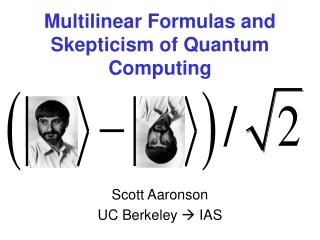Download PresentationMultilinear Formulas and Skepticism of Quantum Computing

# Multilinear Formulas and Skepticism of Quantum Computing - PowerPoint PPT Presentation

Multilinear Formulas and Skepticism of Quantum Computing. Scott Aaronson UC Berkeley  IAS. “We have never seen a physical law valid to over a dozen decimals” (Leonid Levin). Even if QM is right, maybe our complexity model is wrong (Oded Goldreich).I am the owner, or an agent authorized to act on behalf of the owner, of the copyrighted work described.
Download Presentation## Multilinear Formulas and Skepticism of Quantum Computing

An Image/Link below is provided (as is) to download presentation

Download Policy: Content on the Website is provided to you AS IS for your information and personal use and may not be sold / licensed / shared on other websites without getting consent from its author.While downloading, if for some reason you are not able to download a presentation, the publisher may have deleted the file from their server.

- - - - - - - - - - - - - - - - - - - - - - - - - - E N D - - - - - - - - - - - - - - - - - - - - - - - - - -
Presentation Transcript
1. Multilinear Formulas and Skepticism of Quantum Computing Scott Aaronson UC Berkeley  IAS

2. “We have never seen a physical law valid to over a dozen decimals”(Leonid Levin) Even if QM is right, maybe our complexity model is wrong(Oded Goldreich) Is Quantum Computing Fundamentally Impossible? It violates the extended Church-Turing thesis Analogy to analog computing and unit-cost arithmetic

3. DIVIDING LINE “Sure/Shor Separator” Question: If quantum computing is impossible, then what criterion separates the quantum states that suffice for factoring from the states we’ve already seen?

4. YAWN 10000 polarized photons Buckyballs, superconducting coils, … The simplest candidates for such a criterion are nonstarters… Exponentially small amplitudes? Thousands of coherent qubits? Goal of Paper: Provide a formal framework for studying possible Sure/Shor separators

5. AmpP Circuit  Tree P TSH OTree Vidal  MOTree  2 2 1 1 Strict containmentContainmentNon-containment  Classical Key Idea:Complexity classification of pure quantum states

6. Intuitively, once we admit | and | into our set of possible states, we’re almost forced to admit || and |+| as well! But what if we take the closure under a polynomial number of those operations?

7. +   + + |11 |12 |01 |11 |02 |12 Tree size of |: Minimum number of vertices in such a tree representing | Tree states: Infinite families of states {|n}n1 for which TS(|n)=nO(1)

8. If then TS(|) equals the multilinear formula size of f to within a constant factor Minimum size of an arithmetic formula for f(x1,…,xn) involving +, , and complex constants, every node of which computes a multilinear polynomial in x1,…,xn Basic Facts About Tree Size • Many interesting states have polynomial TS1D spin chain, superposition over multiples of 3, … • A random state can’t even be approximated by states with subexponential TS

9. Want to know the proof technique? Go to my talk RAN RAZ Main Result: Tree Size Lower Bound Let C = {x | Axb (mod 2)}, where A is chosen uniformly at random from Then w.h.p. over A, the coset state has tree size n(log n)

10. Significance: Coset states underlie quantum error correction. Our result gives a second reason to prepare them: refuting the hypothesis that “all states in Nature are tree states” Purely classical corollary: First superpolynomial gap between general and multilinear formula size of functions Extensions:Tree size n(log n) is needed even to approximate coset statesCan derandomize, to get an n(log n) lower bound for an explicit coset state

11. But what about states arising in Shor’s algorithm? Conjecture: Let A consist of 5+log(n1/3) uniform random elements of {20,…,2n-1}. Let S consist of all 32n1/3 sums of subsets of A. If a prime p is drawn uniformly from [n1/3,1.1n1.3], then |Sp|  3n1/3/4with probability at least ¾, where Sp= {x mod p : xS} Theorem: Assuming the conjecture, Shor states have tree size n(log n)

12. What’s been done in liquid NMR: (Knill et al. 2001) Non-tree states might already have been observed in solid state! 2D or 3D spin lattices with nearest-neighbor Hamiltonians—similar to “cluster states” 0 0 1 0 1 0 1 1 1 0 0 0 1 1 1 1 0 1 0 0 1 0 0 1 1 Experimental Situation To interpret experiments, would be nice to have explicit tree size lower bounds for small n Key question: What kind of evidence is needed to prove a state’s existence?

13. If a quantum computer is in a tree state at every time step, can it then be simulated classically?Current result: Can be simulated in Open Problems Can we show exponential tree size lower bounds? Do all codeword states have superpolynomial tree size? Relationship between tree size and persistence of entanglement?